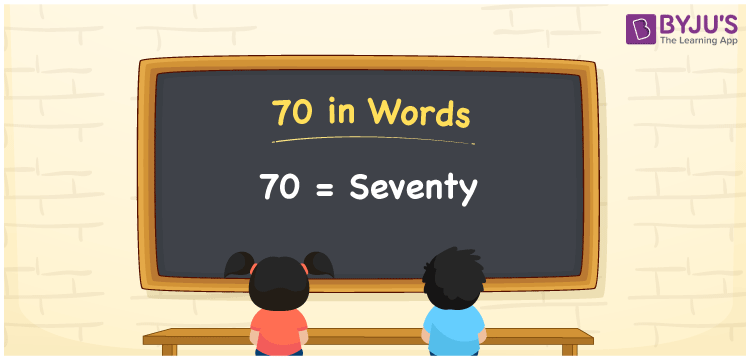# 70 in Words

We can write 70 in words as Seventy. Using a place value chart, we can quickly derive the number 70 in words. If you have 70 pins, then you can say, “I have Seventy pins with me”. Also, 70 is a cardinal number as it denotes some specific quantity.

 70 in words Seventy Seventy in Numbers 70

## 70 in English Words

We generally write numbers in words using the English alphabet. Thus, we can read 70 using the words in English as “Seventy”.## How to Write 70 in Words?

As we can see, 70 is a two-digit number so let’s make a place value chart with two columns as given below.

 Tens Ones 7 0

Here, ones = 0 and tens = 7.

This can be combined as shown below:

7 × Tens + 0 × Ones

= 7 × 10 + 0 × 1

= 70

= Seventy

Therefore, 70 in words = Seventy.

70 is a natural number that is the successor of 69 and the predecessor of 71.

70 in words – Seventy

Is 70 an odd number? – No

Is 70 an even number? – Yes

Is 70 a prime number? – No

Is 70 a composite number? – Yes

Is 70 a perfect square number? – No

Is 70 a perfect cube number? – No

How do you write 70 in Roman numerals? – LXX

### 61 to 70 in Words

 61 in words = Sixty-one 66 in words = Sixty-six 62 in words = Sixty-two 67 in words = Sixty-seven 63 in words = Sixty-three 68 in words = Sixty-eight 64 in words = Sixty-four 69 in words = Sixty-nine 65 in words = Sixty-five 70 in words = Seventy

## Frequently Asked Questions on 70 in Words

Q1

### How to spell 70?

The spelling of 70 in words is Seventy, which means 70 in words can be expressed as Seventy.
Q2

### How do you write Rs. 70 on a cheque?

On a cheque, Rs. 70 can be written as “Seventy rupees only” and we generally mention the amount as Rs. 70/-.
Q3

### Write the value of 60 + 10 in words.

60 + 10 = 70 Sixty + Ten = Seventy Thus, 60 + 10 is equal to Seventy.